Updating search results...

# 42 Results

View
Selected filters:
• PolygonsUnrestricted Use
CC BY
Rating
0.0 stars

Family facing 6th Grade math unit focusing on area and surface area.

Subject:
Geometry
Material Type:
Unit of Study
Provider:
Illustrative Mathematics
05/11/2020Unrestricted Use
CC BY
Rating
0.0 stars

In this unit, students learn to find areas of polygons by decomposing, rearranging, and composing shapes. They learn to understand and use the terms “base” and “height,” and find areas of parallelograms and triangles. Students approximate areas of non-polygonal regions by polygonal regions. They represent polyhedra with nets and find their surface areas.

Subject:
Geometry
Material Type:
Unit of Study
Provider:
Illustrative Mathematics
05/11/2020Unrestricted Use
CC BY
Rating
0.0 stars

This task examines the ways in which the plane can be covered by regular polygons in a very strict arrangement called a regular tessellation. These tessellations are studied here using algebra, which enters the picture via the formula for the measure of the interior angles of a regular polygon (which should therefore be introduced or reviewed before beginning the task). The goal of the task is to use algebra in order to understand which tessellations of the plane with regular polygons are possible.

Subject:
Mathematics
Algebra
Geometry
Material Type:
Activity/Lab
Provider:
Illustrative Mathematics
Provider Set:
Illustrative Mathematics
Author:
Illustrative Mathematics
01/21/2013Conditional Remix & Share Permitted
CC BY-NC-SA
Rating
0.0 stars

In this activity, learners use a hand-made protractor to measure angles they find in playground equipment. Learners will observe that angle measurements do not change with distance, because they are distance invariant, or constant. Note: The "Pocket Protractor" activity should be done ahead as a separate activity (see related resource), but a standard protractor can be used as a substitute.

Subject:
Geometry
Material Type:
Activity/Lab
Provider:
Exploratorium
Author:
Exploratorium
Gordon and Betty Moore Foundation
National Science Foundation
The Exploratorium
12/07/2010Only Sharing Permitted
CC BY-NC-ND
Rating
4.0 stars

This lesson unit is intended to help you assess how well students are able to use geometric properties to solve problems. In particular, it will support you in identifying and helping students who have the following difficulties: Solving problems relating to using the measures of the interior angles of polygons; and solving problems relating to using the measures of the exterior angles of polygons.

Subject:
Geometry
Material Type:
Assessment
Lesson Plan
Provider:
Shell Center for Mathematical Education
Provider Set:
Mathematics Assessment Project (MAP)
04/26/2013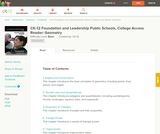Conditional Remix & Share Permitted
CC BY-NC-SA
Rating
0.0 stars

This book is a "flexed" version of CK-12's Basic Geometry that aligns with College Access Geometry and contains embedded literacy supports. It covers the essentials of geometry for the high school student.

Subject:
Geometry
Material Type:
Textbook
Provider:
CK-12 Foundation
Provider Set:
CK-12 FlexBook
Author:
Fauteux, Michael
Zapata, Rosamaria
05/18/2011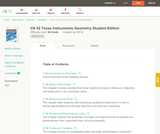Conditional Remix & Share Permitted
CC BY-NC-SA
Rating
0.0 stars

CK-12 Foundation's Geometry FlexBook is a clear presentation of the essentials of geometry for the high school student. Topics include: Proof, Congruent Triangles, Quadrilaterals, Similarity, Perimeter & Area, Volume, and Transformations.

Subject:
Geometry
Material Type:
Textbook
Provider:
CK-12 Foundation
Provider Set:
CK-12 FlexBook
10/06/2009
Remix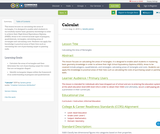Unrestricted Use
CC BY
Rating
0.0 stars

This lesson focuses on calculating the areas of rectangles. It is designed to enable adult students to successfully master basic geometry knowledge in order to achieve their High School Equivalency Diploma (HSED). Areas to be covered include types of polygons, quadrilaterals, rectangles; calculating areas of rectangles and calculating costs. Students can apply this knowledge to practical areas of their lives such as calculating the cost of purchasing carpet or painting walls.

Subject:
Mathematics
Material Type:
Interactive
Lesson Plan
Author:
Sandra James
08/08/2019
Remix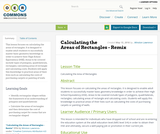Unrestricted Use
CC BY
Rating
0.0 stars

This lesson focuses on calculating the areas of rectangles. It is designed to enable adult students to successfully master basic geometry knowledge in order to achieve their High School Equivalency (HSE). Areas to be covered include types of polygons, quadrilaterals, rectangles; calculating areas of rectangle and calculating costs. Students will apply this knowledge to practical areas of their lives such as calculating the costs of purchasing carpets or painting of walls

Subject:
Mathematics
Material Type:
Interactive
Lesson Plan
Author:
Winston Lawrence
08/08/2019Educational Use
Rating
4.0 stars

In this video segment from Cyberchase, the CyberSquad measures Judge Trudy's land claim by using tarps, fence posts and a grid made with rope.

Subject:
Mathematics
Material Type:
Lecture
Provider:
PBS LearningMedia
Provider Set:
PBS Learning Media: Multimedia Resources for the Classroom and Professional Development
Author:
U.S. Department of Education
WNET
07/07/2008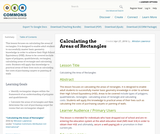Unrestricted Use
CC BY
Rating
0.0 stars

This lesson focuses on calculating the areas of rectangles. It is designed to enable adult students to successfully master basic geometry knowledge in order to achieve their High School Equivalency (HSE). Areas to be covered include types of polygons, quadrilaterals, rectangles; calculating areas of rectangle and calculating costs. Students will apply this knowledge to practical areas of their lives such as calculating the costs of purchasing carpets or painting of walls

Subject:
Mathematics
Material Type:
Interactive
Lesson Plan
Author:
Winston Lawrence
05/05/2016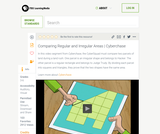Educational Use
Rating
3.0 stars

The CyberSquad proves that the area of Hacker's land is equal to the area of Judge Trudy's land in this video segment from Cyberchase.

Subject:
Mathematics
Material Type:
Lecture
Provider:
PBS LearningMedia
Provider Set:
PBS Learning Media: Multimedia Resources for the Classroom and Professional Development
Author:
U.S. Department of Education
WNET
07/08/2008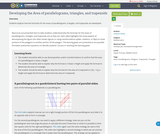Conditional Remix & Share Permitted
CC BY-NC-SA
Rating
0.0 stars

Students explore how the formulas for the areas of parallelograms, triangles, and trapezoids are developed.

Subject:
Mathematics
Material Type:
Activity/Lab
Homework/Assignment
Author:
Heather Bolles
05/13/2020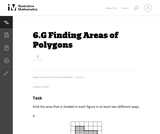Unrestricted Use
CC BY
Rating
0.0 stars

The purpose of this task is to have students work on a sequence of area problems that shows the advantage of increasingly abstract strategies in preparation for developing general area formulas for parallelograms and triangles.

Subject:
Mathematics
Geometry
Material Type:
Activity/Lab
Provider:
Illustrative Mathematics
Provider Set:
Illustrative Mathematics
Author:
Illustrative Mathematics
05/01/2012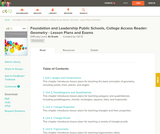Conditional Remix & Share Permitted
CC BY-NC-SA
Rating
0.0 stars

A structured geometry program teacher edition of daily lesson plans and teacher supports to accompany the College Access Reader: Geometry student edition.

Subject:
Geometry
Material Type:
Textbook
Provider:
CK-12 Foundation
Provider Set:
CK-12 FlexBook
Author:
Fauteux, Michael
Zapata, Rosamaria
05/18/2011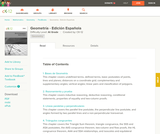Conditional Remix & Share Permitted
CC BY-NC-SA
Rating
0.0 stars

CK-12 Foundation's Geometry FlexBook is a clear presentation of the essentials of geometry for the high school student. Topics include: Proof, Congruent Triangles, Quadrilaterals, Similarity, Perimeter & Area, Volume, and Transformations.

Subject:
Geometry
Material Type:
Textbook
Provider:
CK-12 Foundation
Provider Set:
CK-12 FlexBook
11/23/2010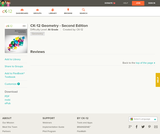Conditional Remix & Share Permitted
CC BY-NC-SA
Rating
0.0 stars

CK-12's Geometry - Second Edition is a clear presentation of the essentials of geometry for the high school student. Topics include: Proofs, Triangles, Quadrilaterals, Similarity, Perimeter & Area, Volume, and Transformations.

Subject:
Geometry
Material Type:
Textbook
Provider:
CK-12 Foundation
Provider Set:
CK-12 FlexBook
Author:
Dirga, Kathryn
Jordan, Lori
11/16/2010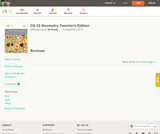Conditional Remix & Share Permitted
CC BY-NC-SA
Rating
0.0 stars

CK-12 Geometry Teacher's Edition covers tips, common errors, enrichment, differentiated instruction and problem solving for teaching CK-12 Geometry Student Edition. The solution and assessment guides are available upon request.

Subject:
Geometry
Material Type:
Textbook
Provider:
CK-12 Foundation
Provider Set:
CK-12 FlexBook
Author:
Kershaw, Jem
Kramer, Melissa
Zwack, Teresa
06/25/2011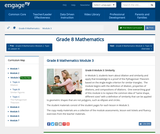Conditional Remix & Share Permitted
CC BY-NC-SA
Rating
0.0 stars

In Module 3, students learn about dilation and similarity and apply that knowledge to a proof of the Pythagorean Theorem based on the Angle-Angle criterion for similar triangles.  The module begins with the definition of dilation, properties of dilations, and compositions of dilations.  One overarching goal of this module is to replace the common idea of same shape, different sizes with a definition of similarity that can be applied to geometric shapes that are not polygons, such as ellipses and circles.

Subject:
Geometry
Material Type:
Module
Provider:
New York State Education Department
Provider Set:
EngageNY
10/17/2013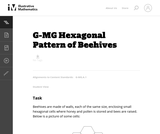Unrestricted Use
CC BY
Rating
0.0 stars

The goal of this task is to use geometry study the structure of beehives. Beehives have a tremendous simplicity as they are constructed entirely of small, equally sized walls. In order to as useful as possible for the hive, the goal should be to create the largest possible volume using the least amount of materials. In other words, the ratio of the volume of each cell to its surface area needs to be maximized. This then reduces to maximizing the ratio of the surface area of the cell shape to its perimeter.

Subject:
Mathematics
Geometry
Material Type:
Activity/Lab
Provider:
Illustrative Mathematics
Provider Set:
Illustrative Mathematics
Author:
Illustrative Mathematics
01/21/2013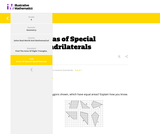Conditional Remix & Share Permitted
CC BY-NC-SA
Rating
0.0 stars

This task asks students to identify which of the six polygons have the same area. Students may complete the task using a variety of techniques including decomposing shapes, using transformations (rotations, reflections, translations) to move one or more parts of the figure to another part to more easily calculate the area, enclosing the polygon inside a larger rectangle and then subtract the areas of the "extra" pieces, etc.

Subject:
Mathematics
Material Type:
Activity/Lab
Diagram/Illustration
Lesson Plan
Provider:
Illustrative Mathematics
05/08/2020Conditional Remix & Share Permitted
CC BY-NC-SA
Rating
0.0 stars

In this activity, learners slide shapes to create unusual tiled patterns. Learners transform a rectangle into a more interesting shape and then make a tessellation by repeating that shape over and over again. Learners will also calculate the area of a rectangle. This activity works best as a "centers" activity.

Subject:
Geometry
Material Type:
Activity/Lab
Provider:
Exploratorium
Author:
Exploratorium
Gordon and Betty Moore Foundation
National Science Foundation
The Exploratorium
12/07/2010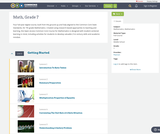Conditional Remix & Share Permitted
CC BY-NC
Rating
0.0 stars

Four full-year digital course, built from the ground up and fully-aligned to the Common Core State Standards, for 7th grade Mathematics. Created using research-based approaches to teaching and learning, the Open Access Common Core Course for Mathematics is designed with student-centered learning in mind, including activities for students to develop valuable 21st century skills and academic mindset.

Subject:
Mathematics
Material Type:
Full Course
Provider:
Pearson
10/06/2016Rating
0.0 stars

Constructions and Angles

Unit Overview

Type of Unit: Concept

Prior Knowledge

Students should be able to:

Use a protractor and ruler.
Identify different types of triangles and quadrilaterals and their characteristics.

Lesson Flow

After an initial exploratory lesson involving a paper folding activity that gets students thinking in general about angles and figures in a context, the unit is divided into two concept development sections. The first section focuses on types of angles—adjacent, supplementary, complementary, and vertical—and how they are manifested in quadrilaterals. The second section looks at triangles and their properties, including the angle sum, and how this affects other figures.

In the first set of conceptual lessons, students explore different types of angles and where the types of angles appear in quadrilaterals. Students fold paper and observe the angles formed, draw given angles, and explore interactive sketches that test many cases. Students use a protractor and ruler to draw parallelograms with given properties. They explore sketches of parallelograms with specific properties, such as perpendicular diagonals. After concluding the investigation of the angle types, students move on to the next set.

In the second set of conceptual development lessons, students focus on triangles. Students again fold paper to create figures and certain angles, such as complementary angles.

Students draw, using a protractor and ruler, other triangles with given properties. Students then explore triangles with certain known and unknown elements, such as the number of given sides and angles. This process starts with paper folding and drawing and continues with exploration of interactive sketches. Students draw conclusions about which cases allow 0, 1, 2, or an infinite number of triangles. In the course of the exploration, students discover that the sum of the measure of the interior angles of a triangle is 180°. They also learn that the sum of the measures of the interior angles of a quadrilateral is 360°. They explore other polygons to find their angle sum and determine if there is a relationship to angle sum of triangles. The exploration concludes with finding the measure of the interior angles of regular polygons and speculating about how this relates to a circle.

Lastly, students solve equations to find unknown angle measures. Using their previous experience, students find the remaining angle measures in a parallelogram when only one angle measure is given. Students also play a game similar to 20 Questions to identify types of triangles and quadrilaterals. Having completed the remaining lessons, students have a four-day Gallery to explore a variety of problems.

The unit ends with a unit assessment.

Subject:
Mathematics
Geometry
Material Type:
Unit of Study
Provider:
Pearson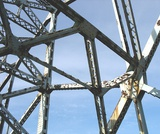Conditional Remix & Share Permitted
CC BY-NC
Rating
0.0 stars

Students learn to classify triangles by the size of the angles and the measures of the sides.Key ConceptsTriangles are polygons with three sides.Scalene triangles have all sides with a different length and all angles with a different measure.Isosceles triangles have two sides with the same length and two angles with the same measure.Equilateral triangles have all sides with the same length and all angles with the same measure.Acute triangles have all angles with a measure less than 90°.Obtuse triangles have one angle with a measure greater than 90°.Right triangles have one angle with a measure of 90°.ELL: Keep in mind that consistency at the beginning is very important as students begin to learn and apply math vocabulary.Goals and Learning ObjectivesExplore conditions that result in triangles.Identify types of triangles based on the measure of the angles or the measures of the sides.

Subject:
Geometry
Material Type:
Lesson Plan
Provider:
Pearson
09/21/2015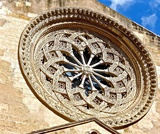Conditional Remix & Share Permitted
CC BY-NC
Rating
0.0 stars

Lesson OverviewStudents explore different polygons by drawing them, measuring angles, and manipulating interactive sketches to find the angle sum for any polygon. Students also explore the angle measures in regular polygons.Key ConceptsThe angle sum in a triangle is 180°. A quadrilateral can be composed of two triangles, so the angle sum of a quadrilateral is 360°.The number of triangles that compose a polygon is two less than the number of sides (angles). The sum of the interior angles in a polygon with n sides is 180° (n – 2).Goals and Learning ObjectivesFind angle sums in polygons.Generalize to find the angle sum for any polygon.Find interior angle measures for regular polygons.

Subject:
Geometry
Material Type:
Lesson Plan
Provider:
Pearson
09/21/2015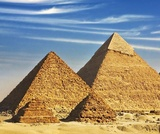Rating
0.0 stars

Zooming In On Figures

Unit Overview

Type of Unit: Concept; Project

Length of Unit: 18 days and 5 days for project

Prior Knowledge

Students should be able to:

Find the area of triangles and special quadrilaterals.
Use nets composed of triangles and rectangles in order to find the surface area of solids.
Find the volume of right rectangular prisms.
Solve proportions.

Lesson Flow

After an initial exploratory lesson that gets students thinking in general about geometry and its application in real-world contexts, the unit is divided into two concept development sections: the first focuses on two-dimensional (2-D) figures and measures, and the second looks at three-dimensional (3-D) figures and measures.
The first set of conceptual lessons looks at 2-D figures and area and length calculations. Students explore finding the area of polygons by deconstructing them into known figures. This exploration will lead to looking at regular polygons and deriving a general formula. The general formula for polygons leads to the formula for the area of a circle. Students will also investigate the ratio of circumference to diameter ( pi ). All of this will be applied toward looking at scale and the way that length and area are affected. All the lessons noted above will feature examples of real-world contexts.
The second set of conceptual development lessons focuses on 3-D figures and surface area and volume calculations. Students will revisit nets to arrive at a general formula for finding the surface area of any right prism. Students will extend their knowledge of area of polygons to surface area calculations as well as a general formula for the volume of any right prism. Students will explore the 3-D surface that results from a plane slicing through a rectangular prism or pyramid. Students will also explore 3-D figures composed of cubes, finding the surface area and volume by looking at 3-D views.
The unit ends with a unit examination and project presentations.

Subject:
Mathematics
Geometry
Material Type:
Unit of Study
Provider:
PearsonConditional Remix & Share Permitted
CC BY-NC
Rating
0.0 stars

Lesson OverviewStudents will compare the formula for the area of a regular polygon to discover the formula for the area of a circle.Key ConceptsThe area of a regular polygon can be found by multiplying the apothem by half of the perimeter. If a circle is thought of as a regular polygon with many sides, the formula can be applied.For a circle, the apothem is the radius, and p is C.A=a(p2)→A=rC2→A=rπd2→A=rπ2r2→A=rπr=πr2 GoalsDerive the formula for the area of a circle.Apply the formula to find the area of circles.SWD: Consider the prerequisite skills for this lesson: understanding and applying the formula for the area of a regular polygon. Students with disabilities may need direct instruction and guided practice with this skill.Students should understand these domain-specific terms:apothemparallelogramderivationheightapproximate (estimate)scatter plotpiperimetercircumferenceIt may be helpful to preteach these terms to students with disabilities.

Subject:
Geometry
Material Type:
Lesson Plan
Provider:
Pearson
09/21/2015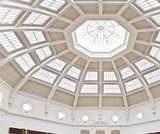Conditional Remix & Share Permitted
CC BY-NC
Rating
0.0 stars

Students find the area of regular polygons, recalling what they already know about the area of geometric shapes and generalizing a formula for any regular polygon.Key ConceptsStudents will recall what they already know about the area of geometric shapes and apply that to find the area of regular polygons. Any regular polygon can be divided into congruent isosceles triangles. If the length from the center to the midpoint of a side (the apothem) is known, the area of the triangles and the area of the polygon can be found. Students will see the similarities between the area of a polygon and derive the formula for the area of a circle.GoalsReview area of triangles, rectangles, and parallelograms.Find the area of regular polygons.Generalize an area formula for any regular polygon.ELL: This lesson offers students a rich opportunity to learn academic vocabulary. Display the new terms in writing somewhere visible in the classroom so that you can refer to them. Allow ELLs to use their bilingual dictionaries to help with understanding the terms. When possible, have ELLs discuss the terms in their language of choice with other ELLs who share the same primary language.

Subject:
Geometry
Material Type:
Lesson Plan
Provider:
Pearson
09/21/2015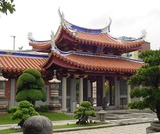Conditional Remix & Share Permitted
CC BY-NC
Rating
0.0 stars

Students critique their work from the Self Check and redo the task after receiving feedback. Students then take a quiz to review the goals of the unit.Key ConceptsStudents understand how to find the area of figures such as rectangles and triangles. They have applied that knowledge to finding the area of composite figures and regular polygons. The area of regular polygons was extended to understand the area of a circle. Students also applied ratio and proportion to interpret scale drawings and redraw them at a different scale.GoalsCritique and revise student work.Apply skills learned in the unit.Understand two-dimensional measurements:Area of composite figures, including regular polygons.Area and circumference of circles.Interpret scale drawings and redraw them at a different scale.SWD: Make sure all students have the prerequisite skills for the activities in this lesson.Students should understand these domain-specific terms:composite figuresregular polygonsareacircumferencescale drawingstwo dimensionalIt may be helpful to preteach these terms to students with disabilities.ELL: As academic vocabulary is reviewed, be sure to repeat it and allow students to repeat after you as needed. Consider writing the words as they are being reviewed. Allow enough time for ELLs to check their dictionaries if they wish.

Subject:
Geometry
Material Type:
Lesson Plan
Provider:
Pearson
09/21/2015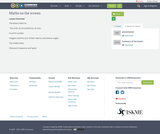Only Sharing Permitted
CC BY-NC-ND
Rating
0.0 stars

The lesson refers to: The circle, its circumference, its areaPi and Fi numberPolygons and the sum of their interior and exterior anglesThe Golden RatioFibonacci’s Sequence and Spiral

Subject:
Computer Science
Graphic Design
Geometry
Ratios and Proportions
Material Type:
Lesson Plan
Author:
Maria Szklarczyk
01/15/2019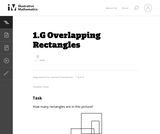Unrestricted Use
CC BY
Rating
4.0 stars

This challenging problem and brainteaser gives students an opportunity to compose and decompose polygons to make rectangles.

Subject:
Mathematics
Geometry
Measurement and Data
Material Type:
Activity/Lab
Provider:
Illustrative Mathematics
Provider Set:
Illustrative Mathematics
Author:
Illustrative Mathematics
08/29/2012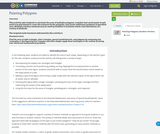Conditional Remix & Share Permitted
CC BY-NC-SA
Rating
0.0 stars

This activity asks students to calculate the area of multiple polygons, consider how much paint would need to be purchased to cover the surfaces by the polygons, and create additional polygons of specified area. Multiple solution methods may be used by students to facilitate whole class discussion of a variety of methods. The targeted math standard addressed by this activity is Standard 6.G.A.1Find the area of right triangles, other triangles, special quadrilaterals, and polygons by composing into rectangles or decomposing into triangles and other shapes; apply these techniques in the context of solving real–world and mathematical problems.

Subject:
Mathematics
Material Type:
Activity/Lab
Author:
Heather Bolles
05/11/2020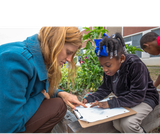Conditional Remix & Share Permitted
CC BY-NC-SA
Rating
0.0 stars

In this lesson the students will use their knowledge of measurement to determine perimeter and find missing lengths when given the total perimeter.

Subject:
Mathematics
Material Type:
Lesson Plan
Author:
Out Teach
07/22/2021Conditional Remix & Share Permitted
CC BY-NC-SA
Rating
0.0 stars

In this lesson the students will use their knowledge of measurement to determine perimeter. Since this is a virtual lesson for at home learning, steps will be included for students to make an 8 inch paper ruler out of notebook paper.

Subject:
Mathematics
Material Type:
Lesson Plan
Author:
Out Teach
07/22/2021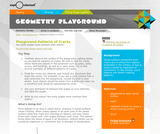Conditional Remix & Share Permitted
CC BY-NC-SA
Rating
0.0 stars

In this math activity, learners observe and sketch cracking patterns in pavement. Learners use a protractor to measure and label the angles of their sketches and conclude if some angles are more common than others.

Subject:
Geometry
Material Type:
Activity/Lab
Provider:
Exploratorium
Author:
Exploratorium
Gordon and Betty Moore Foundation
National Science Foundation
The Exploratorium
12/07/2010Conditional Remix & Share Permitted
CC BY-NC-SA
Rating
0.0 stars

In this activity, learners create angle-measuring devices--protractors--out of paper. Learners follow a series of steps to fold a square sheet of paper into a triangular Pocket Protractor. Learners will practice measuring and identifying the angles of a triangle.

Subject:
Geometry
Material Type:
Activity/Lab
Provider:
Exploratorium
Author:
Exploratorium
Gordon and Betty Moore Foundation
National Science Foundation
The Exploratorium
11/07/2010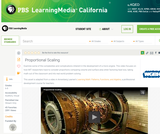Educational Use
Rating
0.0 stars

Learn about the dynamic relationships between a jet engine's heat loss, surface area, and volume in this video adapted from Annenberg Learner's Learning Math: Patterns, Functions, and Algebra.

Subject:
Mathematics
Material Type:
Lesson
Provider:
PBS LearningMedia
Provider Set:
PBS Learning Media Common Core Collection
Author:
U.S. Department of Education
WGBH Educational Foundation
06/18/2012Educational Use
Rating
0.0 stars

This interactive activity adapted from the Wisconsin Online Resource Center challenges you to plan, measure, and calculate the correct amount of roofing material needed to reroof a house.

Subject:
Engineering
Mathematics
Geometry
Material Type:
Interactive
Provider:
PBS LearningMedia
Provider Set:
PBS Learning Media Common Core Collection
Author:
National Science Foundation
WGBH Educational Foundation
12/08/2009Educational Use
Rating
3.0 stars

In this Cyberchase video segment, the CyberSquad learns what happens to the area of a rectangle when the shape enclosed by the perimeter changes.

Subject:
Mathematics
Geometry
Material Type:
Lecture
Provider:
PBS LearningMedia
Provider Set:
PBS Learning Media: Multimedia Resources for the Classroom and Professional Development
Author:
U.S. Department of Education
WNET